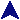Simple (Slow) SortProlog Examples```% The relation permute(X,Y) is satisfied when Y is a permutation of X (or vice
% versa).  The relation var(X) is a builtin which is satisfied when X is
% an unbound variable.  Essentially, we want to evaluate permute when its
% first argument is known, and the second is the result.  The second rule
% simply flips then two when this is not the case.
permute([],[]).
permute(X,Y) :- var(X), permute(Y,X).
permute(L1, [L2H | L2T]) :- not(var(L1)),
append(L1F1, [L2H | L1F2], L1), append(L1F1, L1F2, L1S),
permute(L1S, L2T).

% The relation ordered(X) is satisfied when the members of X are arranged in
% ascending order.
ordered([]).
ordered([_]).
ordered([A,B|C]) :- A =< B, ordered([B|C]).

% Perform sorting straight from the definition.
sorted(X,Y) :- permute(X,Y), ordered(Y).
```